Groundwater Contaminant Transport. 3-D Pulse (Slug) Injection

Groundwater. Chemical injected instantly into an aquifer. Advection, dispersion. Compute concentration, mass, distance.

Register to enable groundwater "Calculate" button.

 Concentration, C (mg/l): Injected Mass, M (kg): Time, t (day): Solve for Concentration Solve for Mass injected Solve for x Solve for y Solve for z Distance, x (m): One dimensional dispersion Two dimensional dispersion Three dimensional dispersion Distance, y (m): Clayey Soil (sets K, n, ne) Silty Soil (sets K, n, ne) Sandy Soil (sets K, n, ne) Gravelly Soil (sets K, n, ne) User enters K, n, ne Distance, z (m): www.LMNOeng.com Dispersivity in x, ax (m): Concentration in mg/l or ppm Concentration in ug/l or ppb Concentration in kg/m3 Dispersivity in y, ay (m): Mass in kg Mass in pounds (mass) Mass in slugs Dispersivity in z, az (m): Time in seconds Time in minutes Time in hours Time in days Time in years Diffusion Coeff, D* (m2/s): Distances in cm Distances in m Distances in km Distances in inch Distances in feet Distances in miles Hydraulic Cond, K (cm/s): Dispersivities in mm Dispersivities in cm Dispersivities in m Dispersivities in inch Dispersivities in feet Hydraulic Grad, -dh/dx (m/m): Diff. and Disp. Coeff. in cm2/s Diff. and Disp. Coeff. in m2/s Diff. and Disp. Coeff. in ft2/s Total Porosity, n (%): Hydraulic Conductivity in cm/s Hydraulic Conductivity in m/s Hydraulic Conductivity in m/day Hydraulic Conductivity in ft/s Hydraulic Conductivity in gal/day/ft2 Effective Porosity, ne (%): Hydraulic Gradient in m/m (ft/ft) Hydraulic Gradient in m/km Hydraulic Gradient in inch/mile Hydraulic Gradient in ft/mile Pore Water Velocity, V (m/s): Velocity in cm/s Velocity in m/s Velocity in m/day Velocity in m/yr Velocity in ft/s Velocity in ft/day Velocity in ft/yr Velocity in mile/hr Dispersion Coeff in x, Dx (m2/s): © 2015 LMNO Engineering, Dispersion Coeff in y, Dy (m2/s): Research, and Software, Ltd. Dispersion Coeff in z, Dz (m2/s): Plume centerline, Vt (m):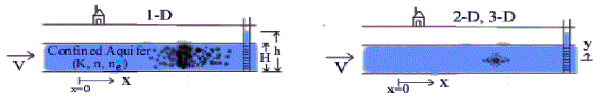Groundwater units: cm=centimeter, ft=foot, g=gram, gal=U.S. gallon, gpd=U.S. gallon per day, hr=hour, kg=kilogram, km=kilometer, l=liter, lb=pound, m=meter, mg=milligram, min=minute, mm=millimeter, ppm=part per million (by mass), ppb=part per billion (by mass), s=second, yr=year, ug=microgram

Topics on groundwater page:   Introduction    Equations    Application    Variable Definitions   Property Data    Error Messages    References

Groundwater Introduction                        Top of Page
This groundwater calculation simulates one, two, or three-dimensional transport of a chemical in a confined groundwater aquifer. It is also valid for transport in an unconfined groundwater aquifer if the head gradient (dh/dx) is nearly constant. The groundwater calculation simulates instantaneous injection of a chemical having a mass M. The groundwater calculation solves for concentration at whatever time and distances are desired by the user. The groundwater calculation also can back-calculate mass or distances.

The groundwater calculation includes advection and dispersion. Advection is chemical movement via groundwater flow due to the groundwater hydraulic (i.e. head) gradient. Dispersion causes spreading of the contaminant. If there were no dispersion, all of the contaminant would travel at the groundwater velocity. With dispersion, some chemical travels faster and some slower than the mean velocity; the chemical "spreads out." In two and three-dimensional dispersion, the chemical additionally disperses upward (and downward) and laterally, respectively, relative to the groundwater plume centerline. The maximum concentration occurs at x=Vt, y=0, and z=0. If you solve for x, y, or z, the groundwater calculation provides two solutions since the plume spreads forward and backward (in the x-direction), up and down (in the y-direction), and in and out (in the z-direction).

Groundwater Equations                         Top of Page

Groundwater Governing Equations
The governing equations for one, two, and three - dimensional chemical transport in groundwater with advection and dispersion are (Bear, 1972):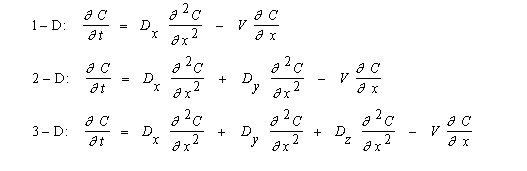Groundwater Boundary Conditions and Solutions
Initially the groundwater aquifer has C=0 everywhere. Then at t=0 and x=0, a chemical slug of mass, M, is injected instantaneously in the groundwater. If M is injected uniformly across a groundwater aquifer's width and height, then there is dispersion only in the x-direction (one-dimensional dispersion). If M is injected uniformly across the groundwater aquifer's width, then dispersion occurs in the x and y directions (two-dimensional dispersion). If M is injected at a point in the groundwater, then dispersion occurs in all three dimensions x, y, and z. The following solutions have been coded in our groundwater calculation (Bear, 1972):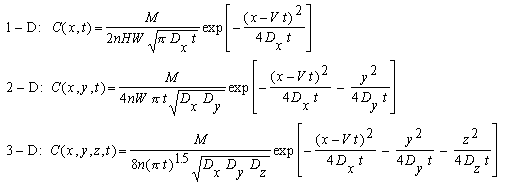where: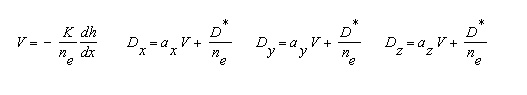Groundwater Application                        Top of Page
The following graph was developed to demonstrate effects of three-dimensional dispersion in a sandy groundwater aquifer as predicted by the calculation. The following data were used:      (Click for variable definitions)
M = 100 kg,  t = 1000 days,  y = z = 0,  ay = az = ax /10.
K = 0.001 cm/s,  n = 35%,  ne = 25%,  dh/dx = -0.007 m/m,   D* = 1.0x10-9 m2/s.

Therefore, V = 2.8x10-7 m/s,  and Vt = 24.192 m.

Figure 1.  Concentration profile at 1000 days for an injection of 100 kg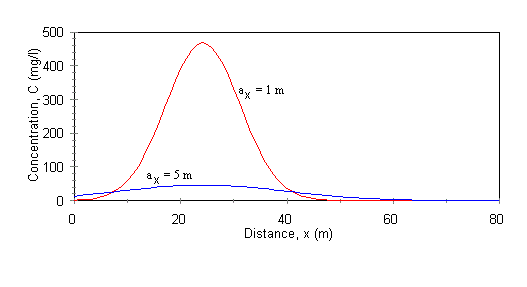Groundwater Variable Definitions   Units: [L]=Length, [M]=Mass, [T]=Time                        Top of Page
The variables used on this groundwater web page are:
ax , ay , az = Dispersivities in x, y, and z directions [L]. Varies from 0.1 to 100 m. Field and laboratory tests have indicated that a varies with the scale of the groundwater test. Large scale groundwater tests have higher a than small lab column tests. An approximate value for ax is 0.1 times the scale of your system (Fetter, 1993). If you are simulating groundwater contaminant transport in a 1 m long laboratory column, then ax~0.1 m. However, if you are simulating transport in a large groundwater aquifer greater than 1 km in extent, then use ax~100 m. ay and az are approximately ax /10 (Javandel et al., 1984, p. 12).
C = Chemical concentration [M/L3].
dh/dx = Groundwater hydraulic (or head) gradient [L/L]. Since dh/dx is negative, we ask you to enter -dh/dx so that you can enter a positive number for convenience. You determine dh/dx from two head measurements using the equation, dh/dx = (h2-h1)/(x2-x1).
Dx , Dy , Dz = Dispersion coefficients in x, y, and z directions [L2/T]. The equation D=a V + D*/ne shown above is adapted from Ingebritsen and Sanford (1998).
D* = Molecular diffusion coefficient [L2/T]. Varies somewhat for different chemicals but a typical value to use is 1.0x10-9 m2/s (Fetter, 1993).
H = Groundwater aquifer height [L]. User enters if one-dimensional dispersion.
K = Hydraulic conductivity of aquifer [L/T].
M = Chemical mass injected [M].
n = Total porosity of soil [%]. (Void volume/total volume) x 100%.
ne = Effective porosity [%]. Porosity through which flow can occur. A thin film of water bound to soil particles by capillary forces does not move through the aquifer. ne is always ≤ n.
t = Time [T]. Time at which C is to be computed.
V = Pore water velocity in x-direction [L/T]. Also known as groundwater velocity.
W = Aquifer width [L]. User enters if one or two-dimensional dispersion.
x, y, z = Distances [L]. Distances at which to compute C. x is the direction of groundwater flow. y is the vertical distance from the centerline of the plume. z is lateral distance (distance into "the computer monitor") from the plume centerline.

Groundwater Property Data                        Top of Page
The following are tables of groundwater hydraulic conductivity, total porosity, and effective porosity. Parameter values have been compiled from a variety of sources such as Freeze and Cherry (1979), Hillel (1982), and Sanders (1998). The values used in the groundwater calculation are typical numbers within the ranges given below.

Table of Soil Properties

 Soil Type Hydraulic Conductivity K (cm/s) Total Porosity n (%) Effective Porosity ne (%) Clayey 10-9 - 10-6 40-60 0-5 Silty 10-7 - 10-3 35-50 3-20 Sandy 10-5 - 10-1 20-50 10-35 Gravelly 10-1 - 102 25-40 12-30

Error Messages given by groundwater calculation                          Top of Page
"Cannot have 1D and Solve for y or z ." No computations. For one-dimensional dispersion, the chemical plume is uniformly distributed across the groundwater aquifer's height and width. y and z are not variables, so they cannot be computed.

"Cannot have 2D and Solve for z ." No computations. For two-dimensional dispersion, the chemical plume is uniformly distributed across the groundwater aquifer's width. z is not a variable, so it cannot be computed.

"H, W must be > 0." No computations. This error message will only appear in one-dimensional dispersion. Groundwater aquifer height and width must be entered, and they must be positive.

"W must be > 0." No computations. This error message will only appear in two-dimensional dispersion. Groundwater aquifer width must be entered, and it must be positive.

"Infeasible input." No computations. This error message will only appear if x, y, or z is being computed. This message will be shown if the ratio of mass to concentration entered is too low to be physically achievable.

"Certain inputs must be ≥ 0." No computations. C, D*, dh/dx, K, M, n, ne , and t must all be ≥ 0 if entered. x, y, and z can be positive, negative, or zero.

"n and ne must be ≤ 100%." No computations. Total porosity and effective porosity cannot exceed 100%.

"ne must be ≤ n." No computations. Effective porosity cannot exceed total porosity.

Groundwater References                       Top of Page
Bear, J. 1972. Dynamics of Fluids in Porous Media. American Elsevier Pub. Co.

Fetter, C. W. 1993. Contaminant Hydrogeology. Macmillan Pub. Co.

Freeze, R. A. and J. A. Cherry. 1979. Groundwater. Prentice Hall, Inc.

Hillel, D. H. 1982. Introduction to Soil Physics. Academic Press, Inc.

Ingebritsen, S. E. and W. E. Sanford. 1998. Groundwater in Geologic Processes. Cambridge University Press.

Javandel, I, C. Doughty, and C. F. Tsang. 1984. Groundwater Transport: Handbook of Mathematical Models. American Geophysical Union.

Sanders, L. L. 1998. A Manual of Field Hydrogeology. Prentice Hall, Inc.

LMNO Engineering, Research, and Software, Ltd.
7860 Angel Ridge Rd.   Athens, Ohio 45701  USA   Phone: (740) 592-1890
LMNO@LMNOeng.com    http://www.LMNOeng.com

To:

Related Calculations:

1-D Step injection with advection, dispersion, retardation